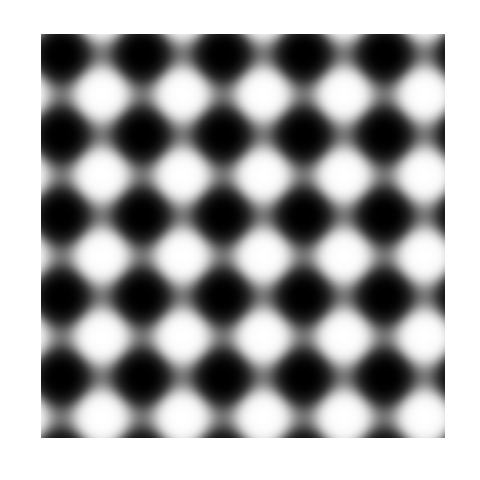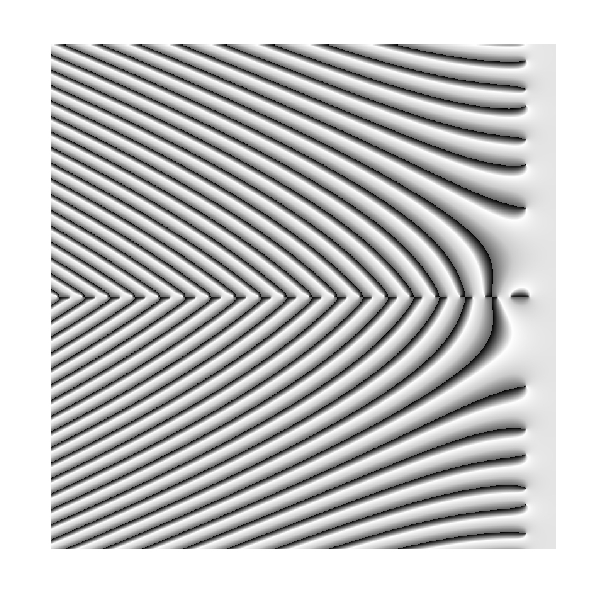# Trigonometric Pattern Design

Triangles are my favorite shape, three points where two lines meet (Tessellate, Alt-J)

Inspired by recurrence plots and by the Gauss error function, I have done the following plots. The first one represents the recurrence plot of$f\left ( x \right )= sec\left ( x \right )$ where distance between points is measured by Gauss error function:This one is the same for$f\left ( x \right )= tag\left ( x \right )$And this one represents$latex f\left ( x \right )= sin\left ( x \right )$I like them: they are elegant, attractive and easy to make. Try your own functions. One final though: the more I use magrittr package, the more I like it. This is the code for the first plot.

library("magrittr")
library("ggplot2")
library("pracma")
RecurrencePlot = function(from, to, col1, col2) {
opt = theme(legend.position  = "none",
panel.background = element_blank(),
axis.ticks       = element_blank(),
panel.grid       = element_blank(),
axis.title       = element_blank(),
axis.text        = element_blank())
seq(from, to, by = .1) %>% expand.grid(x=., y=.) %>%
ggplot( ., aes(x=x, y=y, fill=erf(sec(x)-sec(y)))) + geom_tile() +
RecurrencePlot(from = -5*pi, to = 5*pi, col1 = "black", col2= "white")


# The Zebra Of Riemann

Mathematics is the art of giving the same name to different things (Henri Poincare)

Many surveys among experts point that demonstration of the Riemann Hypothesis is the most important pending mathematical issue in this world. This hypothesis is related to Riemann zeta function, which is supossed to be zero only for those complex whose real part is equal to 1/2 (this is the conjecture of Riemann itself). Confirming the conjecture would imply deep consequences in prime numbers teory and also in our knowledge of their properties. Next plot represents the argument of zeta function over complex with real part between -75 and 5 (x axis) and imaginary part between -40 and 40 (y axis). An explanation of this kind of graph can be found here. Does not it remind you of something?Solving the hypothesis of Riemann is one of the seven Clay Mathematics Institute Millennium Prize Problems. Maybe zebras keep the secret to solve it in their skins.

This is the code to plot the graph:

require(pracma)
z=outer(seq(-75, 5, by =.1),1i*seq(-40, 40, by =.1),'+')
z=apply(z, c(1,2), function(x) Arg(zeta(x)))
opt=theme(legend.position="none",
panel.background = element_blank(),
panel.grid = element_blank(),
axis.ticks=element_blank(),
axis.title=element_blank(),
axis.text =element_blank())
z=data.frame(expand.grid(x=seq(ncol(z)), y=seq(nrow(z))), z=as.vector(z))
require(ggplot2)
ggplot(z, aes(x=x, y=y, color=z)) + geom_tile() + scale_colour_gradientn(colours=c("black","white", "gray80", "gray40")) + opt